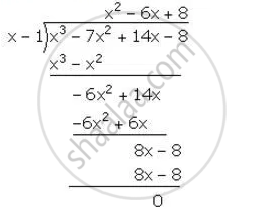Share

Show that (X – 1) is a Factor of X3 – 7x2 + 14x – 8. Hence, Completely Factorise the Given Expression. - ICSE Class 10 - Mathematics

ConceptFactorising a Polynomial Completely After Obtaining One Factor by Factor Theorem

Question

Show that (x – 1) is a factor of x3 – 7x2 + 14x – 8. Hence, completely factorise the given expression.

Solution

let f(x)=x^3-7x^2+14x-8

f(1)=(1)^3-7(1)^2+14(1)-8=1-7+14-8=0

Hence, (x-1) is a factor of f(x)∴ x^3-7x^2+14x-8=(x-1) (x^2-6x+8)

= (x-1)(x^2-2x-4x+8)

= (x-1)[x(x-2)-4(x-2)]

=(x-1) (x-2) (x-4)

Is there an error in this question or solution?

APPEARS IN

Solution Show that (X – 1) is a Factor of X3 – 7x2 + 14x – 8. Hence, Completely Factorise the Given Expression. Concept: Factorising a Polynomial Completely After Obtaining One Factor by Factor Theorem.
S